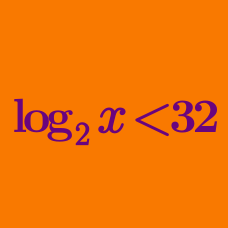Algebra

Logarithmic Inequalities: Level 2 Challenges

It is well known that $\ln 2 < \ln 3$. Which of the following is bigger:

$[ \ln ( \ln 2 ) ] ^2 \text { or } [ \ln ( \ln 3) ]^2 ?$

Find the range of positive value of $x$ such that $\log_3 (x+7) < \log_9(x^2+77)$ is fulfilled?

Which of the following logarithms is greater?$\color{#20A900}{\log _{9}{71}} ~~~~\text{or}~~~~\color{#3D99F6}{\log _{8}{61}}$

$\ln\left(2x^{2} - 3x + 8\right) \le \dfrac{\ln\left(x^{4}+4x^{3} + 8x^{2} + 8x + 4\right)}{2}$

Find the product of all integer values of $x$ which satisfy the inequality above.

True or false:

For $1, the inequality $\log_{10} (x+99) > x$ is satisfied.

×